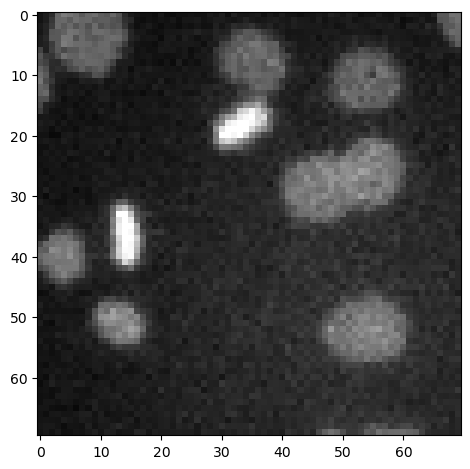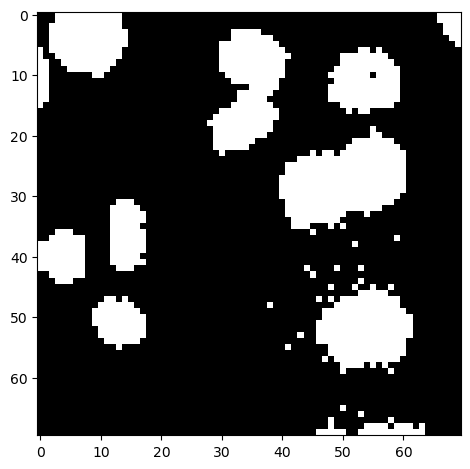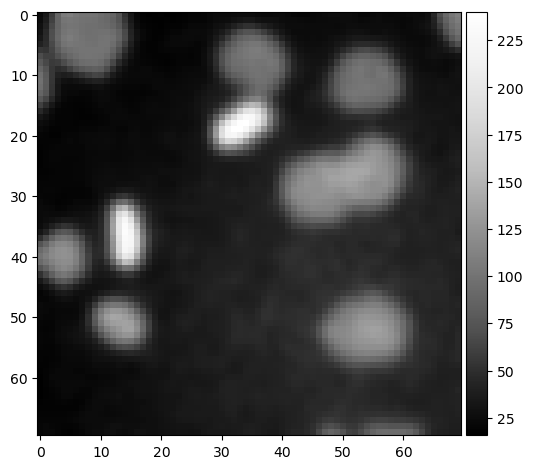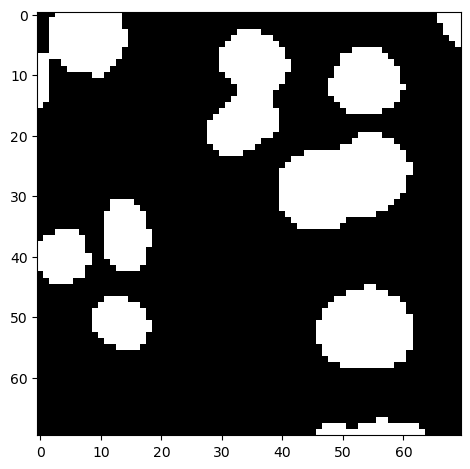# Image Binarization#

When binarizing an image, we produce an image that has only two values: True and False. They may also contain the value 0, e.g. for background, and any other value for foreground.

import numpy as np
from skimage.filters import gaussian


We use this example image of nuclei.

image_nuclei = imread('../../data/mitosis_mod.tif')

imshow(image_nuclei)

<matplotlib.image.AxesImage at 0x1dcf6b5dbe0>## Image Thesholding#

The most common binarization technique is thresholding. We apply a threshold to determine which pixel lie above a certain pixel intensity and which are below.

image_binary = image_nuclei > 60

imshow(image_binary)

<matplotlib.image.AxesImage at 0x1dcf6bb09a0>## Improving binarization results#

Sometimes, binarization results appear pixelated. This can be improved by applying a filter before thresholding the image.

image_denoised = gaussian(image_nuclei, sigma=1, preserve_range=True)
imshow(image_denoised, cmap='Greys_r')

C:\Users\haase\mambaforge\envs\bio39\lib\site-packages\skimage\io\_plugins\matplotlib_plugin.py:150: UserWarning: Float image out of standard range; displaying image with stretched contrast.
lo, hi, cmap = _get_display_range(image)

<matplotlib.image.AxesImage at 0x1dcf6c48640>image_binary2 = image_denoised > 60

imshow(image_binary2)

<matplotlib.image.AxesImage at 0x1dcf7d61040>